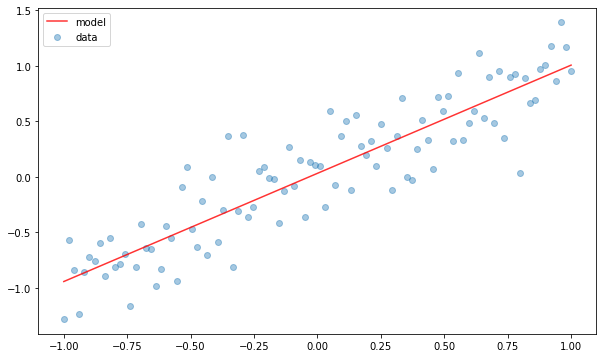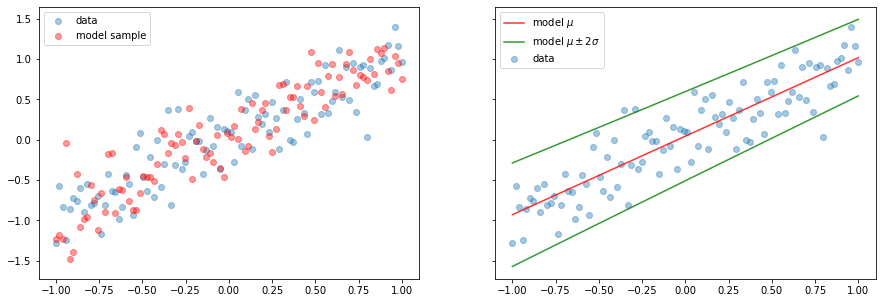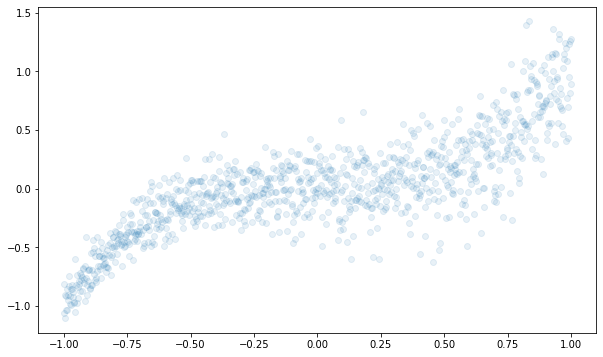## Packages

import tensorflow as tf
import tensorflow_probability as tfp

import numpy as np
import matplotlib.pyplot as plt

tfd = tfp.distributions
tfpl = tfp.layers

plt.rcParams['figure.figsize'] = (10, 6)

print("Tensorflow Version: ", tf.__version__)
print("Tensorflow Probability Version: ", tfp.__version__)

Tensorflow Version:  2.5.0
Tensorflow Probability Version:  0.13.0


## Overview

### Model with DistributionLambda Layer

from tensorflow.keras.models import Sequential
from tensorflow.keras.layers import Dense

model = Sequential([
Dense(16, activation='relu', input_shape=(2, )),
Dense(2), # (batch_size, 2)
tfpl.DistributionLambda(
# Note that ellipse means the batch size
lambda t: tfd.Independent(tfd.Normal(loc=t[..., :1],
scale=tf.math.softplus(t[..., 1:])))
)
])


Note that tf.math.softplus is a smooth version of the relu activation function. And it's strictly positive and injective. Currently, we didn't set reinterpreted_batch_ndims argument in Independent Layer, and default operation with no argument is transfering all batch dimensions except the first into the event shape.

And its output is kind of single random variable with normal distribution.

### Model with IndependentNormal Layer

This is the case of using IndependentNormal layer instead of DistributionLambda layer

import tensorflow_probability as tfp
tfd = tfp.distributions
tfpl = tfp.layers

model = Sequential([
Dense(16, activation='relu', input_shape=(2, )),
Dense(2),
tfpl.IndependentNormal(1), # Event shape of 1
])


Note that, IndependentNormal layer is inherit object from a DistributionLambda layer.

We can also use it for two dimensional multivariate normal with diagonal covariance matrix.

import tensorflow_probability as tfp
tfd = tfp.distributions
tfpl = tfp.layers

model = Sequential([
Dense(16, activation='relu', input_shape=(2, )),
Dense(4), # Now it has 4 dense node, since two for the mean and two for the standard deviation
tfpl.IndependentNormal(2), # Event shape of 2
])


Or we can define the event shape with rank (> 1).

import tensorflow_probability as tfp
tfd = tfp.distributions
tfpl = tfp.layers

model = Sequential([
Dense(16, activation='relu', input_shape=(2, )),
Dense(8),
tfpl.IndependentNormal([2, 2]), # Event shape of [2, 2]
])


General form is like this:

event_shape = 2
model = Sequential([
Dense(16, activation='relu', input_shape=(2, )),
Dense(tfpl.IndependentNormal.params_size(event_shape)),
tfpl.IndependentNormal(event_shape)
])


param_size API returns the parameters needed for this probabilistic layer with specific event shape.

If you want to get sample tensor from this Probabilistic Layer, we can use same approach mentioned from previous post. Use convert_to_tensor_fn argument.

event_shape = 2
model = Sequential([
Dense(16, activation='relu', input_shape=(2, )),
Dense(tfpl.IndependentNormal.params_size(event_shape)),
tfpl.IndependentNormal(event_shape,
convert_to_tensor_fn=tfd.Distribution.sample)
])


### Classification Example with MLE

from tensorflow.keras.models import Sequential
from tensorflow.keras.layers import Flatten, Dense, Conv2D, MaxPooling2D
import tensorflow_probability as tfp
tfd = tfp.distributions
tfpl = tfp.layers

num_classes = 10

model = Sequential([
Conv2D(16, (3, 3), activation='relu', input_shape=(32, 32, 3)),
MaxPooling2D((3, 3)),
Flatten(),
Dense(64, activation='relu'),
Dense(tfpl.OneHotCategorical.params_size(num_classes)),
tfpl.OneHotCategorical(num_classes)
])

model.compile(loss=lambda y_true, y_pred: -y_pred.log_prob(y_true))
model.fit(X_train, y_train, epochs=20)


## Tutorial

from tensorflow.keras.models import Sequential
from tensorflow.keras.layers import Dense
from tensorflow.keras.losses import MeanSquaredError
from tensorflow.keras.optimizers import RMSprop


### Create data

The data you'll be working with is artifically created from the following equation: $$y_i = x_i + \frac{3}{10}\epsilon_i$$ where $\epsilon_i \sim N(0, 1)$ are independent and identically distributed.

X_train = np.linspace(-1, 1, 100)[:, np.newaxis]
y_train = X_train + 0.3 * np.random.randn(100)[:, np.newaxis] # with Gaussian Noise

plt.scatter(X_train, y_train, alpha=0.4)
plt.xlabel('x')
plt.ylabel('y')
plt.show()### Baseline - Deterministic linear regression with MSE loss

model = Sequential([
Dense(units=1, input_shape=(1, ))
])

model.compile(loss=MeanSquaredError(), optimizer=RMSprop(learning_rate=0.005))
model.fit(X_train, y_train, epochs=200, verbose=False)

<tensorflow.python.keras.callbacks.History at 0x7f3c33399ad0>
model.summary()

Model: "sequential"
_________________________________________________________________
Layer (type)                 Output Shape              Param #
=================================================================
dense (Dense)                (None, 1)                 2
=================================================================
Total params: 2
Trainable params: 2
Non-trainable params: 0
_________________________________________________________________

plt.scatter(X_train, y_train, alpha=0.4, label='data')
plt.plot(X_train, model.predict(X_train), color='red', alpha=0.8, label='model')
plt.legend(loc='best')
plt.show()x = np.array([])
y = model(x)
y

<tf.Tensor: shape=(1, 1), dtype=float32, numpy=array([[0.03125635]], dtype=float32)>

The output of model should contain the uncertainty, not deterministic value. The Deterministic model doesn't have this property, and it always show same value with same input (no uncertainty). In other words, this model fails to capture the aleatoric uncertainty, which is, even if I knew the x value and best fit of this regression, I cannot sure what the next point is.

### Probabilistic linear regression with both user-defined and learned variance

# Parameterized Independent normal distribution with specific mean and 1 std (user defined)
model = Sequential([
Dense(units=1, input_shape=(1, )),
tfpl.DistributionLambda(lambda t: tfd.Independent(tfd.Normal(loc=t, scale=1)))
], name='probabilistic_regression')

model.summary()

Model: "probabilistic_regression"
_________________________________________________________________
Layer (type)                 Output Shape              Param #
=================================================================
dense_1 (Dense)              (None, 1)                 2
_________________________________________________________________
distribution_lambda (Distrib multiple                  0
=================================================================
Total params: 2
Trainable params: 2
Non-trainable params: 0
_________________________________________________________________

def nll(y_true, y_pred):
return -y_pred.log_prob(y_true)

model.compile(loss=nll, optimizer=RMSprop(learning_rate=0.005))
model.fit(X_train, y_train, epochs=100, verbose=False)

<tensorflow.python.keras.callbacks.History at 0x7f3c308a4790>
y_model = model(x)
y_model

<tfp.distributions.Independent 'probabilistic_regression_distribution_lambda_Independentprobabilistic_regression_distribution_lambda_Normal' batch_shape= event_shape= dtype=float32>

The output is the distribution object, not tensor object.

y_model = model(X_train)
y_sample = y_model.sample()
y_hat = y_model.mean()
y_std = y_model.stddev()

y_hat_m2std = y_hat - 2 * y_std
y_hat_p2std = y_hat + 2 * y_std

fig, (ax1, ax2) = plt.subplots(1, 2, figsize=(15, 5), sharey=True)
ax1.scatter(X_train, y_train, alpha=0.4, label='data')
ax1.scatter(X_train, y_sample, alpha=0.4, color='red', label='model sample')
ax1.legend(loc='best')

ax2.scatter(X_train, y_train, alpha=0.4, label='data')
ax2.plot(X_train, y_hat, color='red', alpha=0.8, label='model $\mu$')
ax2.plot(X_train, y_hat_m2std, color='green', alpha=0.8, label='model $\mu \pm 2 \sigma$')
ax2.plot(X_train, y_hat_p2std, color='green', alpha=0.8)
ax2.legend(loc='best')
plt.show()Currently, all sampled data from model lies in $2\sigma$ from normal distribution. But it seems to wide besides original dataset distribution. Since we defined standard deviation of normal distribution manually, it make sense.

In order to handle it, we also need to parameterize standard deviation. Note that standard deviation should be positive, its value needs to wrap up softplus.

# Parameterized Independent normal distribution with specific mean and std
model = Sequential([
Dense(units=2, input_shape=(1, )),
tfpl.DistributionLambda(lambda t: tfd.Independent(tfd.Normal(
loc=t[..., :1], scale=tf.math.softmax(t[..., 1:]))))
], name='probabilistic_regression_scale')

model.summary()

Model: "probabilistic_regression_scale"
_________________________________________________________________
Layer (type)                 Output Shape              Param #
=================================================================
dense_2 (Dense)              (None, 2)                 4
_________________________________________________________________
distribution_lambda_1 (Distr multiple                  0
=================================================================
Total params: 4
Trainable params: 4
Non-trainable params: 0
_________________________________________________________________


Or we can build the sample model with IndependentNormal Layer.

event_shape = 1

model = Sequential([
Dense(units=tfpl.IndependentNormal.params_size(event_shape), input_shape=(1, )),
tfpl.IndependentNormal(event_shape)
], name='probabilistic_regression_scale')

model.summary()

Model: "probabilistic_regression_scale"
_________________________________________________________________
Layer (type)                 Output Shape              Param #
=================================================================
dense_3 (Dense)              (None, 2)                 4
_________________________________________________________________
independent_normal (Independ multiple                  0
=================================================================
Total params: 4
Trainable params: 4
Non-trainable params: 0
_________________________________________________________________

model.compile(loss=nll, optimizer=RMSprop(learning_rate=0.005))
model.fit(X_train, y_train, epochs=100, verbose=False)

<tensorflow.python.keras.callbacks.History at 0x7f3c308ab650>
y_model = model(X_train)
y_sample = y_model.sample()
y_hat = y_model.mean()
y_std = y_model.stddev()

y_hat_m2std = y_hat - 2 * y_std
y_hat_p2std = y_hat + 2 * y_std

fig, (ax1, ax2) = plt.subplots(1, 2, figsize=(15, 5), sharey=True)
ax1.scatter(X_train, y_train, alpha=0.4, label='data')
ax1.scatter(X_train, y_sample, alpha=0.4, color='red', label='model sample')
ax1.legend(loc='best')

ax2.scatter(X_train, y_train, alpha=0.4, label='data')
ax2.plot(X_train, y_hat, color='red', alpha=0.8, label='model $\mu$')
ax2.plot(X_train, y_hat_m2std, color='green', alpha=0.8, label='model $\mu \pm 2 \sigma$')
ax2.plot(X_train, y_hat_p2std, color='green', alpha=0.8)
ax2.legend(loc='best')
plt.show()model(x).sample()

<tf.Tensor: shape=(1, 1), dtype=float32, numpy=array([[-0.1540999]], dtype=float32)>
model(x).sample()

<tf.Tensor: shape=(1, 1), dtype=float32, numpy=array([[0.04947972]], dtype=float32)>

### Probabilistic linear regression with nonlinear learned mean & variance

Let's change the data to being nonlinear: $$y_i = x_i^3 + \frac{1}{10}(2 + x_i)\epsilon_i$$ where $\epsilon_i \sim N(0, 1)$ are independent and identically distributed.

X_train = np.linspace(-1, 1, 1000)[:, np.newaxis]
y_train = np.power(X_train, 3) + 0.1 * (2 + X_train) * np.random.randn(1000)[:, np.newaxis]

plt.scatter(X_train, y_train, alpha=0.1)
plt.show()event_shape = 1

model = Sequential([
Dense(units=8, input_shape=(1, ), activation='sigmoid'),
Dense(tfpl.IndependentNormal.params_size(event_shape)),
tfpl.IndependentNormal(event_shape)
])

model.compile(loss=nll, optimizer=RMSprop(learning_rate=0.01))
model.summary()

Model: "sequential_1"
_________________________________________________________________
Layer (type)                 Output Shape              Param #
=================================================================
dense_4 (Dense)              (None, 8)                 16
_________________________________________________________________
dense_5 (Dense)              (None, 2)                 18
_________________________________________________________________
independent_normal_1 (Indepe multiple                  0
=================================================================
Total params: 34
Trainable params: 34
Non-trainable params: 0
_________________________________________________________________

model.fit(X_train, y_train, epochs=200, verbose=False)
model.evaluate(X_train, y_train)

32/32 [==============================] - 0s 815us/step - loss: -0.1479

-0.14790906012058258
y_model = model(X_train)
y_sample = y_model.sample()
y_hat = y_model.mean()
y_std = y_model.stddev()
y_hat_m2std = y_hat - 2 * y_std
y_hat_p2std = y_hat + 2 * y_std

fig, (ax1, ax2) = plt.subplots(1, 2, figsize=(15, 5), sharey=True)
ax1.scatter(X_train, y_train, alpha=0.4, label='data')
ax1.scatter(X_train, y_sample, alpha=0.4, color='red', label='model sample')
ax1.legend(loc='best')

ax2.scatter(X_train, y_train, alpha=0.4, label='data')
ax2.plot(X_train, y_hat, color='red', alpha=0.8, label='model $\mu$')
ax2.plot(X_train, y_hat_m2std, color='green', alpha=0.8, label='model $\mu \pm 2 \sigma$')
ax2.plot(X_train, y_hat_p2std, color='green', alpha=0.8)
ax2.legend(loc='best')
plt.show()